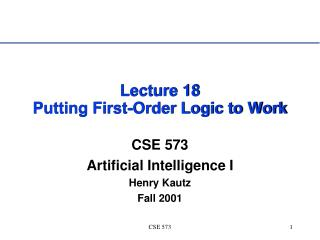DownloadDownload PresentationLecture 18 Putting First-Order Logic to Work

# Lecture 18 Putting First-Order Logic to Work

Télécharger la présentation## Lecture 18 Putting First-Order Logic to Work

- - - - - - - - - - - - - - - - - - - - - - - - - - - E N D - - - - - - - - - - - - - - - - - - - - - - - - - - -
##### Presentation Transcript

1. Lecture 18Putting First-Order Logic to Work CSE 573 Artificial Intelligence I Henry Kautz Fall 2001 CSE 573

2. Applications of FOL • Proving Theorems in Mathematics • Proving Programs Correct • Programming • Ontologies – what kids of things makes up the world? • Planning – how does the world change over time? CSE 573

3. Theorem Proving • Mathematics • Small number of axioms • Deep results • In 1933, E. V. Huntington presented the following basis for Boolean algebra: • x + y = y + x. [commutativity] • (x + y) + z = x + (y + z). [associativity] • n(n(x) + y) + n(n(x) + n(y)) = x. [Huntington equation] • Shortly thereafter, Herbert Robbins conjectured that the Huntington equation can be replaced with a simpler one: • n(n(x + y) + n(x + n(y))) = x. [Robbins equation] • Algebras satisfying commutativity, associativity, and the Robbins equation became known as Robbins algebras. Question: is every Robbins algebra Boolean? • Solved October 10, 1996, by the theorem prover EQP • Open question for 63 years! • William McCune, Argonne National Laboratory • 8 days on an RS/6000 processor CSE 573

4. Theorem Proving • Program Verification • Huge number of axioms • Tedious (but vital!) results • J. Strother Moore (Boyer-Moore Theorem Prover) • Correctness of the AMD5k86 Floating-Point Division: If p and d are double extended precision floating-point numbers (d /= 0) and mode is a rounding mode specifying a rounding style and target format of precision n not exceeding 64, then the result delivered by the K5 microcode is p/d rounded according to mode. CSE 573

5. Logic Combinatory logic Logic calculi Henkin models Mathematics Set theory Graph theory Algebra Boolean algebra Robbins algebra Left distributive Lattices Groups Rings General algebra Number theory Topology Analysis Geometry Field theory Category theory Computer science Computing theory Knowledge representation Natural Language Processing Planning Software creation Software verification Engineering Hardware creation Hardware verification Social sciences Management Syntactic Puzzles Miscellaneous The TPTP (Thousands of Problems for Theorem Provers) Problem Library CSE 573

6. Progress • Automated theorem proving “stalled” in 1980’s • Recent resurgence • Massive memory, speed • Code sharing via web • you can download a program to do your homework! • Integration of propositional reasoning techniques into FOL theorem proving • clever heuristics for grounding formulas CSE 573

7. Programming in Logic • Prolog – FOL as a programming logic • FO Horn clauses • still Turing complete! • restricted form of resolution theorem proving • Idea: Predicate = Program • Function symbols = way to build data structures [ 1, 2, 3 ] = cons(1,cons(2,cons(3,nil))) •  X, T . member(X, cons(X, T)) •  X, Y, T . (member(X, T)  member(X, cons(Y,T))) • member(X, [X|T]). • member(X, [Y|T]) :- member(X, T). • query: member(3, [1, 2, 3, 4]) returns true CSE 573

8. Prolog: Computing Values • append([], L, L). • append([H|L1], L2, [H|L3]) :- append(L1,L2,L3). • queries: • append([1,2],[3,4],[1,2,3,4]) returns true • append([1,2],[3,4],X) returns X = [1,2,3,4] • append([1,2],Y,[1,2,3,4]) returns Y=[3,4] CSE 573

9. Deductive Databases • Datalog: • Facts = DB relations (tables) • Rules = Prolog without function symbols • Decidable, but PTIME-complete • salary_by_name(X,Y) :- ssn(X,N) & salary_by_ssn(N,Y). CSE 573

10. Ontologies • on·tol·o·gy n. The branch of metaphysics that deals with the nature of being. • AI definition: a set of axioms that describe some aspect of the world in terms of types of objects and relationships between objects. CSE 573

11. Example Ontology: Categories anything abstract physical position emotion machine animate animal happiness robot human CSE 573

12. Example Ontology: Subtypes anything abstract physical position emotion machine animate animal happiness robot human  x . (anything(x) (physical(x)  abstract(x)))  x . (robot(x) machine(x)) CSE 573

13. Example Ontology: Relations anything abstract physical location position emotion machine animate animal experience happiness robot human  x . (physical(x)  y . (position(y) location(x,y)) CSE 573

14. Example Ontology: Instances anything abstract physical location position emotion machine animate animal experience happiness robot human robot(R2D2)location(R2D2, X24Y99Z33)  position(X24Y99Z33) R2D2 CSE 573

15. Why Formalize Ontologies? • Knowledge exchange and reuse • Common syntax not enough • How do the your meanings relate to my meanings? • Is Bill Gate’s meaning of “expensive” the same as mine? • What to do when we have different ways of conceptualizing the world? • Eskimo’s words for snow CSE 573

16. Categories in Dyirbal, an aboriginal language of Australia • Bayi: men, kangaroos, possums, bats, most snakes, most fishes, some birds, most insects, the moon, storms, rainbows, boomerangs, some spears, etc. • Balan: women, anything connected with water or fire, bandicoots, dogs, platypus, echidna, some snakes, some fishes, most birds, fireflies, scorpions, crickets, the stars, shields, some spears, some trees, etc. • Balam: all edible fruit and the plants that bear them, tubers, ferns, honey, cigarettes, wine, cake. • Bala: parts of the body, meat, bees, wind, yamsticks, some spears, most trees, grass, mud, stones, noises, language, etc. CSE 573

17. Representing Change CSE 573